### Examples of drawing scientific diagrams with Asymptote in IPython notebook¶

These examples are taken from the gallery on Asymptote's website. We start by installing the asymptote IPython magics remotely.

In :
# Install the IPython magic extension
%install_ext http://raw.github.com/azjps/ipython-asymptote/master/asymptote.py

Installed asymptote.py. To use it, type:

In :
# Load the extension


#### Examples¶

In :
%%asy
size(250);

real a=3;
real b=4;
real c=hypot(a,b);

transform ta=shift(c,c)*rotate(-aCos(a/c))*scale(a/c)*shift(-c);
transform tb=shift(0,c)*rotate(aCos(b/c))*scale(b/c);

picture Pythagorean(int n) {
picture pic;
fill(pic,scale(c)*unitsquare,1/(n+1)*green+n/(n+1)*brown);
if(n == 0) return pic;
picture branch=Pythagorean(--n);
return pic;
}



Out: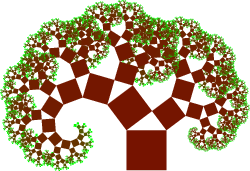Using the -f --outformat argument, the output format of the image can be changed. By default the image is output as a png. Asymptote may require additional configurations or third party programs (ImageMagick) for additional formats.

In :
%%asy -f png
import graph3;
import palette;
import contour3;
size(400);

real f(real x, real y, real z) {
return cos(x)*sin(y)+cos(y)*sin(z)+cos(z)*sin(x);
}

surface sf=surface(contour3(f,(-2pi,-2pi,-2pi),(2pi,2pi,2pi),12));

currentlight=nolight;

draw(sf,render(merge=true));


Out: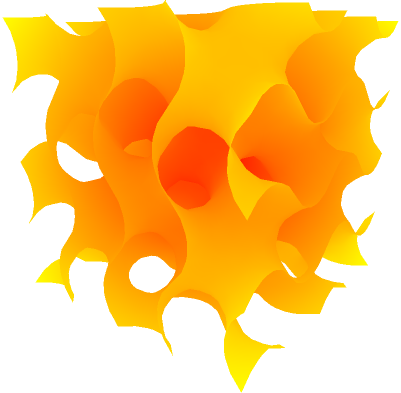A local asymptote code file can also be compiled and displayed if its specified as the argument to an asy file:

In :
!head fin.asy

// See http://asymptote.sourceforge.net/gallery/fin.asy

import three;
import palette;

int N = 26;
real[] C = array(N,0);
real[][] A = new real[N][N];
for(int i = 0; i < N; ++i)
for(int j = 0; j < N; ++j)

In :
%asy fin.asy


Out: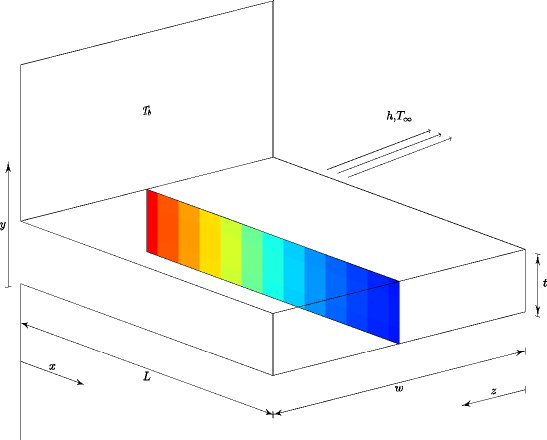We can also interactively append asymptote code to these files:

In :
%%asy fin.asy
label("I added this new label right now!", (2.0,0,0), NE);


Out: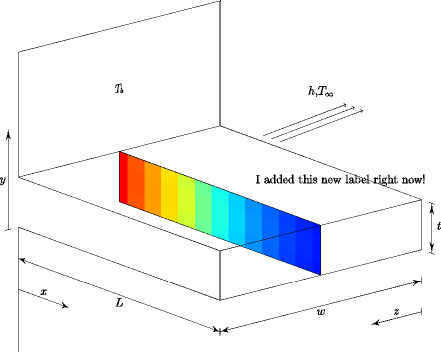To save the intermediate .asy code file and output image, use the -r --root argument.

In :
%%asy --root cube
import three;
currentprojection=orthographic(5,4,2, center=true);

size(5cm);
size3(3cm,5cm,8cm);

draw(unitbox);
dot(unitbox, red);

label("$O$", (0,0,0), NW);
label("(1,0,0)", (1,0,0), S);
label("(0,1,0)", (0,1,0), E);
label("(0,0,1)", (0,0,1), Z);


Out: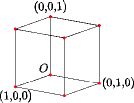In :
!head cube.asy

import three;
currentprojection=orthographic(5,4,2, center=true);

size(5cm);
size3(3cm,5cm,8cm);

draw(unitbox);
dot(unitbox, red);

label("$O$", (0,0,0), NW);